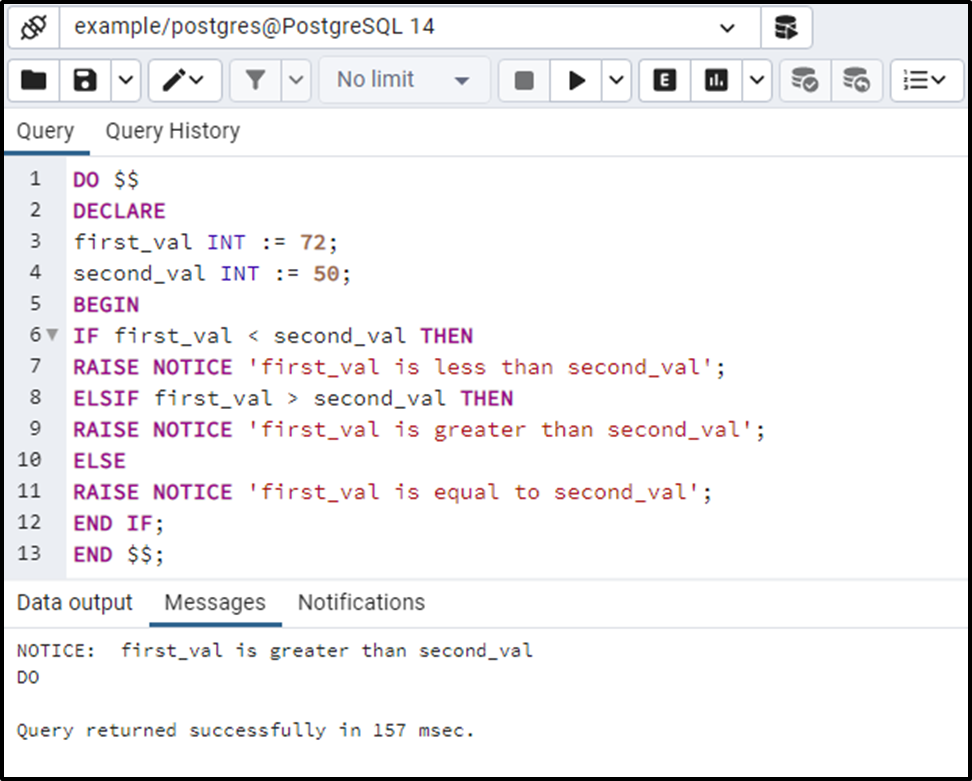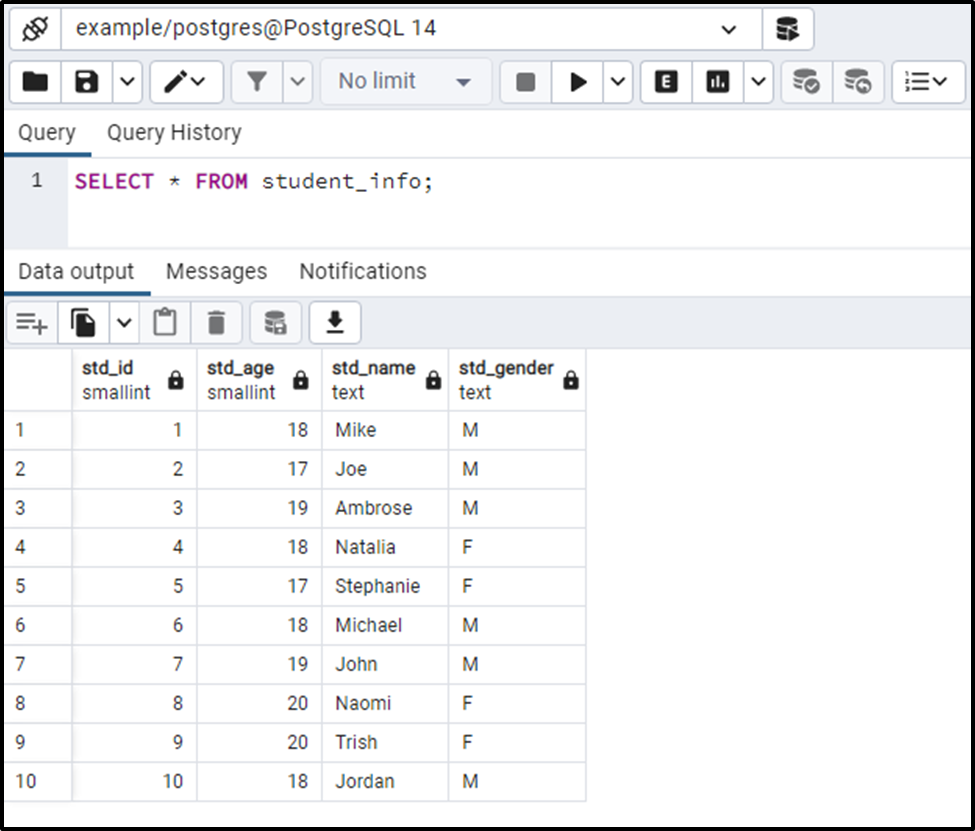##### PostgreSQL Else If Statement With Examples

PostgreSQL offers several decision-making statements such as IF, IF-THEN-ELSE, IF-THEN-ELSIF, etc. All these decision-driven statements are used to control the flow of the SQL statements based on specific criteria. In Postgres, the IF and IF-THEN-ELSE statements evaluate only one condition; however, the IF-THEN-ELSIF statement evaluates several conditions.

This write-up will discuss the working of the IF-THEN-ELSIF statement through practical examples. So, let’s start.

How Does the ELSE IF Statement Work in PostgreSQL?

IF-THEN-ELSIF is one of the decision-driven statements that evaluate several conditions.

• The IF THEN ELSIF statement checks/evaluates each condition one by one.
• When a condition becomes true, all the statements associated with that condition will get executed, and the rest of the conditions will be skipped.
• If none of the specified conditions retrieve a true value, then the statements associated with the else part will get executed.

Syntax

The below snippet elaborates the syntax of the IF-THEN-ELSIF statement:

```IF condition_1 THEN
Statements;  //gets executed only if condition_1 retrieves true.
ELSIF condition_2 THEN
Statements;  //gets executed only if condition_2 retrieves true.
...
ELSIF condition_n THEN
Statements; //gets executed only if condition_n retrieves true.
ELSE
Statements; //gets executed only if all the provided conditions retrieve false.
END IF;```

Example #1: How to Use IF-THEN-ELSIF Statement in Postgres?
Let’s create two variables and assign them some random values:

```DO \$\$
DECLARE
first_val INT := 72;
second_val INT := 50;
BEGIN
IF first_val < second_val THEN
RAISE NOTICE 'first_val is less than second_val';
ELSIF first_val > second_val THEN
RAISE NOTICE 'first_val is greater than second_val';
ELSE
RAISE NOTICE 'first_val is equal to second_val';
END IF;
END \$\$;```

In this example, we created two variables named first_val and second_val. We assigned them integer values. Afterward, we utilized conditional statements to compare their values:

• In the if statement, we checked whether the first_val < second_val; if yes, then show the message “first_val is less than second_val”.
• Else if the first_val > second_val; then return “first_val is greater than second_val”.
• Else raise a notice “first_val is equal to the second_val”.The output shows that the condition specified in the ELSIF part retrieves a true value, so the statement associated with the ELSIF part gets executed.

Example #2: How to Use ELSIF Statement on Table’s Data?

We have created a table named student_info and inserted the following records into it:

`SELECT * FROM student_info;`Now we will specify the following five scenarios in the control statements:

• If std_age <= 18 and std_gender = M then show “Teenage Male”.
• If std_age <= 18 and std_gender = F then show “Teenage Female”.
• If std_age > 18 and std_gender = M then show “Adult Male”.
• If std_age > 18 and std_gender = F then show “Adult Female”.
• If none of the above-given conditions return true, then show a notice "student with the specified id doesn't exist in the student_info table".
```DO \$\$
DECLARE
student_data student_info%rowtype;
BEGIN
SELECT * FROM student_info
INTO student_data
WHERE std_id = 3;
IF student_data.std_age <=18 AND student_data.std_gender= 'M' THEN
RAISE NOTICE 'Teenage Male';
ELSIF student_data.std_age > 18 AND student_data.std_gender= 'M' THEN
ELSIF student_data.std_age <=18 AND student_data.std_gender= 'F' THEN
RAISE NOTICE 'Teenage Female';
ELSIF student_data.std_age >18 AND student_data.std_gender= 'F' THEN